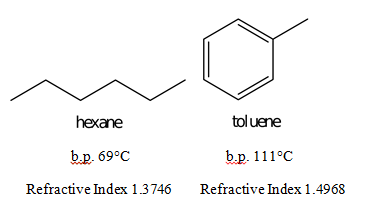# Simple Distillation: Separation of Hexane and Toluene

Simple Distillation: Separation of Hexane and Toluene
By: Jessica Hauger

Abstract: The purpose of this experiment is to separate a mixture of hexane and toluene by collecting fractions through simple distillation. Because hexane’s boiling point is about 69°C and the boiling point of toluene is 111°C, the two compounds distill at different times. The distillation took place in a simple distilling apparatus. The refractive index was measured using an Abbé refractometer. After collecting only two fractions and recording the refractive index of each fraction, the percentage of toluene and hexane could be calculated. The first fraction, taken from 60°C to 68°C, contained 89.85% hexane and 10.15% toluene. The other fraction that was collected consisted of 7.28% hexane and 92.72% toluene. Because the boiling point of hexane is so much smaller than that of toluene, the second fraction consisted mostly of toluene.

Structure:Procedure: Reference pages 10 through 11 titled Simple Distillation: Separation of Hexane and Toluene in the Organic Chemistry Lab Manual posted to blackboard by Dr. Kelly. Omit the collection of a second distillate. Add the collection of the third fraction through cooling of the remaining liquid and collection of that.

Results:

 Fraction Temperature Range Refractive Index Percentages 60°C-68°C 1.3871 89.85% Hexane, 10.15% Toluene None, taken from left over liquid 1.4879 7.28% Hexane, 92.72% Toluene

Calculations:

Refractive Index = X(1.3746) + (1-X)(1.4968)

Refractive Index = 1.4968 – 0.1222X

First Fraction:

1.3871=1.4968 – 0.1222X

X = .8985

89.58% hexane

1-0.8985 = 0.1015

10.15% toluene

Second Fraction:

1.4879 = 1.4968 – 0.1222X

X = .0728

7.28% hexane

1 – .0728 = .9272

92.72% toluene

Boiling point difference:

111ºC – 69ºC = 42ºC

Conclusions and Discussions:

Simple distillation, when used to separate two substances, is used mostly when those two substances have boiling points approximately 40ºC apart. The difference between toluene, with a boiling point of 111ºC and hexane, with a boiling point of 69ºC, is 42ºC, making these two substances most easily separated by simple distillation. Distilled between 60ºC and 68ºC, the first fraction collected contained 89.85% hexane and 10.15% toluene. This concurs with the boiling points of the two substances because hexane has such a lower boiling point than toluene. Because of this, most of the toluene was distilled in the first fraction. After attempting to heat and distill the rest of the solution for some time, the most efficient method to get another fraction was to cool the mixture and extract the rest of the solution into a new clean test tube. This fraction contained 7.28% hexane and 92.72% toluene. Basically, the hexane had mostly distilled in the first fraction. Compared to the results of my colleagues, my results were fairly normal, perhaps even more precise than others, with the exclusion of the inability to collect a second fraction.

The main error in this procedure was the inability of the solution to heat up above 68ºC. An overly swift heating of the mixture for the first fraction could have contributed to this. Because the hexane had mostly distilled in the first fraction, the second fraction obtained through cooling and direct extraction without distillation was almost completely toluene. These results were not abnormal, despite not obtaining the second or third fractions correctly, if at all.

• Share